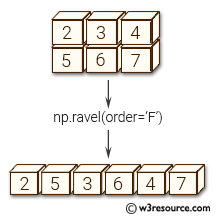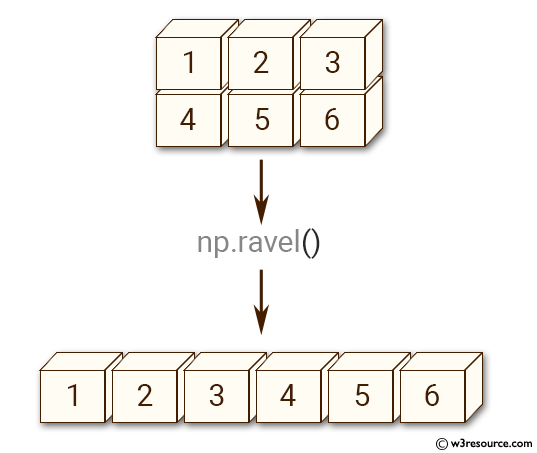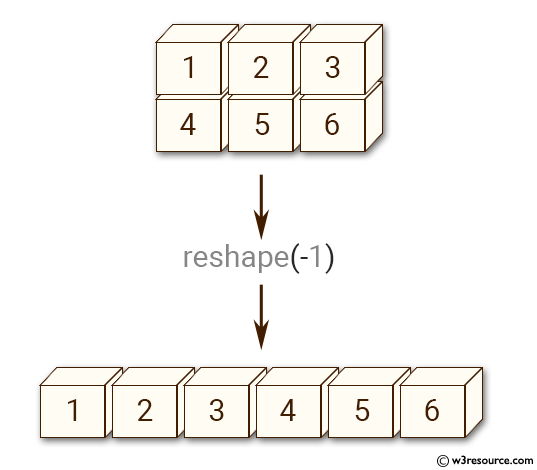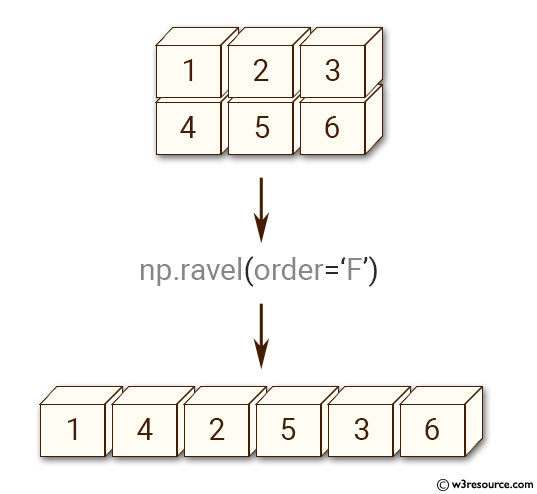# NumPy Array manipulation: ravel() function

## numpy.ravel() function

The ravel() function is used to create a contiguous flattened array.
A 1-D array, containing the elements of the input, is returned. A copy is made only if needed.
As of NumPy 1.10, the returned array will have the same type as the input array. (for example, a masked array will be returned for a masked array input)

Syntax:

`numpy.ravel(a, order='C')`Version: 1.15.0

Parameter:

Name Description Required /
Optional
a Input array. The elements in a are read in the order specified by order, and packed as a 1-D array. Required
order The elements of a are read using this index order. ‘C’ means to index the elements in row-major, C-style order, with the last axis index changing fastest, back to the first axis index changing slowest. ‘F’ means to index the elements in column-major, Fortran-style order, with the first index changing fastest, and the last index changing slowest. Note that the ‘C’ and ‘F’ options take no account of the memory layout of the underlying array, and only refer to the order of axis indexing. ‘A’ means to read the elements in Fortran-like index order if a is Fortran contiguous in memory, C-like order otherwise. ‘K’ means to read the elements in the order they occur in memory, except for reversing the data when strides are negative. By default, ‘C’ index order is used. Ooptional

Return value:

y : array_like - y is an array of the same subtype as a, with shape (a.size,). Note that matrices are special cased for backward compatibility, if a is a matrix, then y is a 1-D ndarray.

Example-1: numpy.ravel() function

``````>>> import numpy as np
>>> x = np.array([[1, 2, 3], [4, 5, 6]])
>>> print(np.ravel(x))
[1 2 3 4 5 6]
``````

Pictorial Presentation:Example-2: numpy.ravel() function

``````>>> import numpy as np
>>> x = np.array([[1, 2, 3], [4, 5, 6]])
>>> print(x.reshape(-1))
[1 2 3 4 5 6]
>>> print(np.ravel(x, order='F'))
[1 4 2 5 3 6]a.ravel
``````

Pictorial Presentation:Example-3: numpy.ravel() function

``````>>> import numpy as np
>>> a = np.array([[2,3,4], [5,6,7]])
>>> print(np.ravel(a, order='F'))
[2 5 3 6 4 7]
>>> print(np.ravel(a.T))
[2 5 3 6 4 7]
>>> print(np.ravel(a.T, order='A'))
[2 3 4 5 6 7]
>>> a = np.arange(2)[::-1]; a
array([1, 0])
>>> a.ravel(order='C')
array([1, 0])
>>> a.ravel(order='K')
array([1, 0])
>>> a.ravel(order='C')
array([1, 0])
>>> a = np.arange(12).reshape(2,3,2).swapaxes(1,2); a
array([[[ 0,  2,  4],
[ 1,  3,  5]],

[[ 6,  8, 10],
[ 7,  9, 11]]])
>>> a.ravel(order='C')
array([ 0,  2,  4,  1,  3,  5,  6,  8, 10,  7,  9, 11])
>>> a.ravel(order='K')
array([ 0,  1,  2,  3,  4,  5,  6,  7,  8,  9, 10, 11])
``````

Python - NumPy Code Editor:

Previous: Changing array shape reshape()
Next: ndarray.flat()

﻿